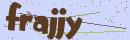# Laravel多表查询优化

2020-02-28 18:59:27

```public function detail()
{
return \$this->where(...)
->with('user');
}

public function user()
{
return \$this->belongsTo('AppUser');
}```

```\$article_id = 1;	// 假设文章 ID 为 1

// 获取到所有评论
\$model_comment = new Comment();
\$rows_comment = \$model_comment->get_comment_by_article_id(\$article_id);

// 获取所有评论的用户
\$array_user_id = array_column(\$rows_comment, 'user_id');
\$model_user = new User();
\$rows_user = \$model_user->get_all_user_by_id(\$array_user_id);

// 接下来就是设置用户的 ID 作为用户数组 \$rows_user 的下标(Key)
\$new_rows_user = [];
foreach (\$rows_user as \$key => \$value) {
\$new_rows_user[\$value['id']] = \$value;
}
dump(\$new_rows_user);
// 打印可以发现 这个数组的下标(Key)就是用户的 ID
// 要获取某个评论的某个用户信息可以这样：
dump(\$new_rows_user[\$rows_comment['user_id]]);```

```function set_index(\$array, \$key_name = 'id')
{
\$new_array = [];
foreach (\$array as \$key => \$value) {
\$new_array[\$value[\$key_name]] = \$value;
}
return \$new_array;
}```

```function set_index_multidimensional(\$array, \$key_name = 'id')
{
\$new_array = [];
foreach (\$array as \$key => \$value) {
\$new_array[\$value[\$key_name]][] = \$value;
}
return \$new_array;
}```

```foreach (\$rows_article as \$row_article) {
// 查询评论

// 再查用户
}```